knn算法 机器学习_机器学习算法——knn算法评价 - CSDN
• 1-1 机器学习算法分类 一、基本分类： ①监督学习（Supervised learning） 数据集中的每个样本有相应的“正确答案”， 根据这些样本做出 预测， 分有两类： 回归问题和分类问题。 步骤1： 数据集的创建和...
文章目录1-1 机器学习算法分类一、基本分类：①监督学习（Supervised learning）②无监督学习③半监督学习④强化学习小结：2-1 KNN基本流程一、概念：2-2 K值的选择2-3 kd树一、原理：二、构造方法：
1-1 机器学习算法分类
一、基本分类：
①监督学习（Supervised learning）
数据集中的每个样本有相应的“正确答案”， 根据这些样本做出
预测， 分有两类： 回归问题和分类问题。
步骤1： 数据集的创建和分类
步骤2： 训练
步骤3： 验证
步骤4： 使用
（ 1） 回归问题举例
例如： 预测房价， 根据样本集拟合出一条连续曲线。
（ 2） 分类问题举例
例如： 根据肿瘤特征判断良性还是恶性，得到的是结果是“良性”或者“恶性”， 是离散的。
监督学习：从给定的训练数据集中学习出一个函数（模型参数）， 当新的数据到来时，可以根据这个函数预测结果。监督学习的训练集要求包括输入输出，也可以说是特征和目标。训练集中的目标是由人标注的。
PCA和很多deep learning算法都属于无监督学习
②无监督学习
无监督学习：输入数据没有被标记，也没有确定的结果。样本数据类别未知， 需要根据样本间的相似性对样本集进行分类（聚类， clustering）试图使类内差距最小化，类间差距最大化。
实际应用中， 不少情况下无法预先知道样本的标签，也就是说没有训练样本对应的类别，因而只能从原先没有样本标签的样本集开始学习分器设计

有监督学习
无监督学习

样本
必须要有训练集与测试样本。在训练集中找规律，而对测试样本使用这种规律。
没有训练集，只有一组数据，在该组数据集内寻找规律。

目标
方法是识别事物，识别的结果表现在给待识别数据加上了标签。 因此训练样本集必须由带标签的样本组成。
方法只有要分析的数据集的本身，预先没有什么标签。如果发现数据集呈现某种聚集性， 则可按自然的聚集性分类，但不予以某种预先分类标签对上号为目的。

③半监督学习
半监督学习： 即训练集同时包含有标记样本数据和未标记样本数据。
④强化学习
实质是： make decisions问题，即自动进行决策，并且可以做连续决策。
主要包含四个元素： agent， 环境状态， 行动， 奖励；
强化学习的目标就是获得最多的累计奖励。
小结：
监督学习：
In：有标签
Out：有反馈
目的：预测结果
案例：学认字
算法：分类（类别），回归（数字）
无监督学习：
In：无标签
Out：无反馈
目的：发现潜在结构
案例：自动聚类
算法：聚类，降维
半监督学习:
已知：训练样本Data和待分类的类别
未知：训练样本有无标签均可
应用：训练数据量过时，
监督学习效果不能满足需求，因此用来增强效果。
强化学习：
In：决策流程及激励系统
Out：一系列行动
目的：长期利益最大化，回报函数（只会提示你是否在朝着目标方向前进的延迟反映）
案例：学下棋
算法：马尔科夫决策，动态规划
2-1 KNN基本流程
一、概念：
KNN（K Near Neighbor）：k个最近的邻居，即每个样本都可以用它最接近的k个邻居来代表。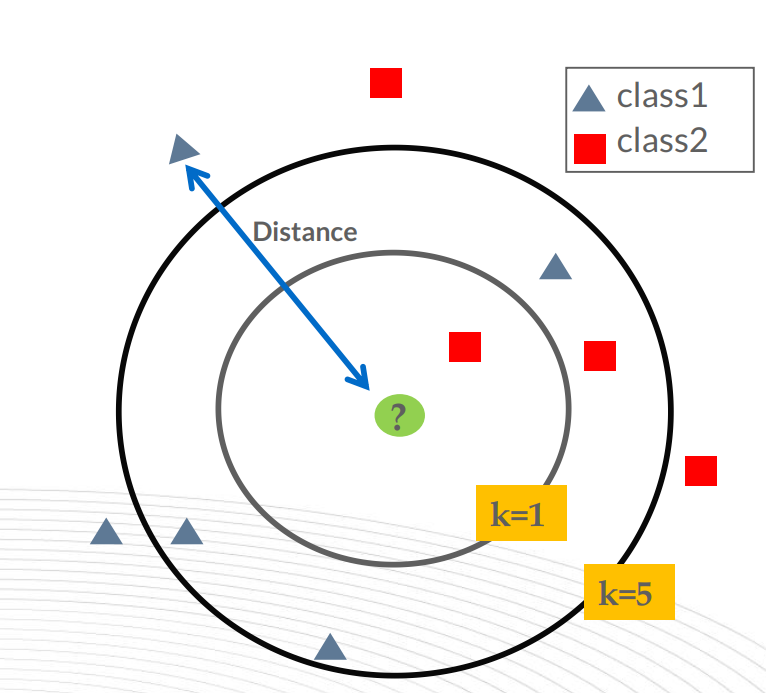最近邻 (k-Nearest Neighbors， KNN) 算法是一种分类算法， 1968年由 Cover和 Hart 提出， 应用场景有字符识别、 文本分类、 图像识别等领域。
该算法的思想是： 一个样本与数据集中的k个样本最相似， 如果这k个样本中的大多数属于某一个类别， 则该样本也属于这个类别。
###二、距离度量
在选择两个实例相似性时，一般使用的欧式距离
Lp距离定义：
$L_p(x_i, x_j) = \left( \sum_{l=1}^{n} | x_i^{(l)} - x_j^{(l)} |^p \right)^{\frac{1}{p}}$
其中$x_i \in \mathbf{R}^n,x_j \in \mathbf{R}^n$, 其中L∞定义为：
$L_\infty(x_i, x_j) = \max_l|x_i^{(l)} - x_j^{(l)}|$
其中p是一个变参数。
当p=1时，就是曼哈顿距离(对应L1范数)
曼哈顿距离对应L1-范数，也就是在欧几里得空间的固定直角坐标系上两点所形成的线段对轴产生的投影的距离总和。例如在平面上，坐标（x1, y1）的点P1与坐标（x2, y2）的点P2的曼哈顿距离为：$|x_1-x_2|+|y_1-y_2|$，要注意的是，曼哈顿距离依赖座标系统的转度，而非系统在座标轴上的平移或映射。
曼哈顿距离：
$L_1=\sum_{k=1}^n|x_{1k}-x_{2k}|$
L1范数表示为:
$L_1定义为|x| = \sum_{i=1}^n| x_i|，其中x = \left[ \begin{matrix} x_1 \\ x_2 \\ \vdots \\ x_n \end{matrix} \right] \in \mathbf{R}^n$
当p=2时，就是欧氏距离（对应L2范数）
最常见的两点之间或多点之间的距离表示法，又称之为欧几里得度量，它定义于欧几里得空间中。n维空间中两个点x1(x11,x12,…,x1n)与 x2(x21,x22,…,x2n)间的欧氏距离
欧氏距离：
$d_{12}=\sqrt{\sum_{k=1}^n(x_1-x_2)^2}$
L2范数：
$L_2定义为|x| = \sqrt{ \sum_{i=1}^n x_i^2 }，其中x = \left[ \begin{matrix} x_1 \\ x_2 \\ \vdots \\ x_n \end{matrix} \right] \in \mathbf{R}^n$
当p→∞时，就是切比雪夫距离
二维平面两点a(x1,y1)与b(x2,y2)间的切比雪夫距离：
$d_{12}=max(|x_1-x_2|,|y_1-y_2|)$
n维空间点a(x11,x12,…,x1n)与b(x21,x22,…,x2n)的切比雪夫距离：
$d_{12}=max(|x_{1i}-x_{2i}|)$
###三、k值选择
如果选择较小的K值，就相当于用较小的邻域中的训练实例进行预测，学习的近似误差会减小，只有与输入实例较近的训练实例才会对预测结果起作用，单缺点是学习的估计误差会增大，预测结果会对近邻的实例点分成敏感。如果邻近的实例点恰巧是噪声，预测就会出错。换句话说，K值减小就意味着整体模型变复杂，分的不清楚，就容易发生过拟合。
如果选择较大K值，就相当于用较大邻域中的训练实例进行预测，其优点是可以减少学习的估计误差，但近似误差会增大，也就是对输入实例预测不准确，K值得增大就意味着整体模型变的简单
**近似误差：**可以理解为对现有训练集的训练误差。
**估计误差：**可以理解为对测试集的测试误差。
近似误差关注训练集，如果k值小了会出现过拟合的现象，对现有的训练集能有很好的预测，但是对未知的测试样本将会出现较大偏差的预测。模型本身不是最接近最佳模型。
估计误差关注测试集，估计误差小了说明对未知数据的预测能力好。模型本身最接近最佳模型。
在应用中，K值一般取一个比较小的数值，通常采用交叉验证法来选取最优的K值。
流程：
1） 计算已知类别数据集中的点与当前点之间的距离
2） 按距离递增次序排序
3） 选取与当前点距离最小的k个点
4） 统计前k个点所在的类别出现的频率
5） 返回前k个点出现频率最高的类别作为当前点的预测分类
优点：
1、简单有效
2、重新训练代价低
3、算法复杂度低
4、适合类域交叉样本
5、适用大样本自动分类
缺点：
1、惰性学习
2、类别分类不标准化
3、输出可解释性不强
4、不均衡性
5、计算量较大
例子如下图：
距离越近，就越相似，属于这一类的可能性就越大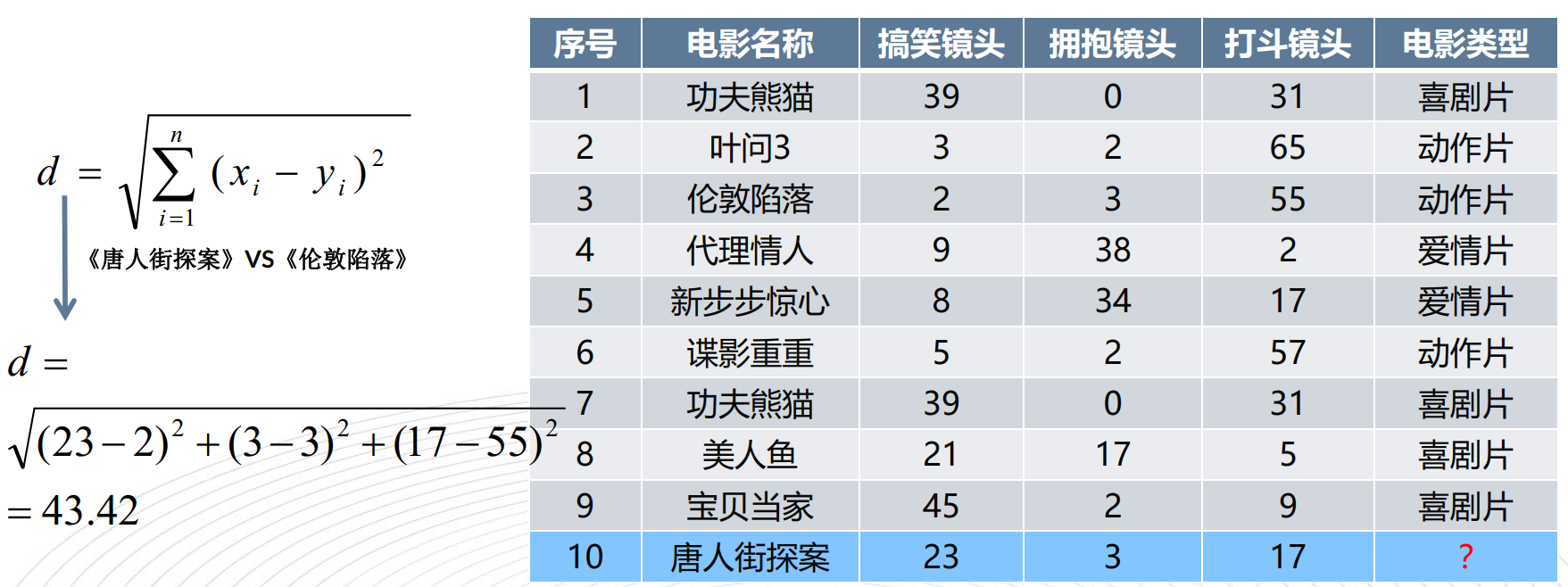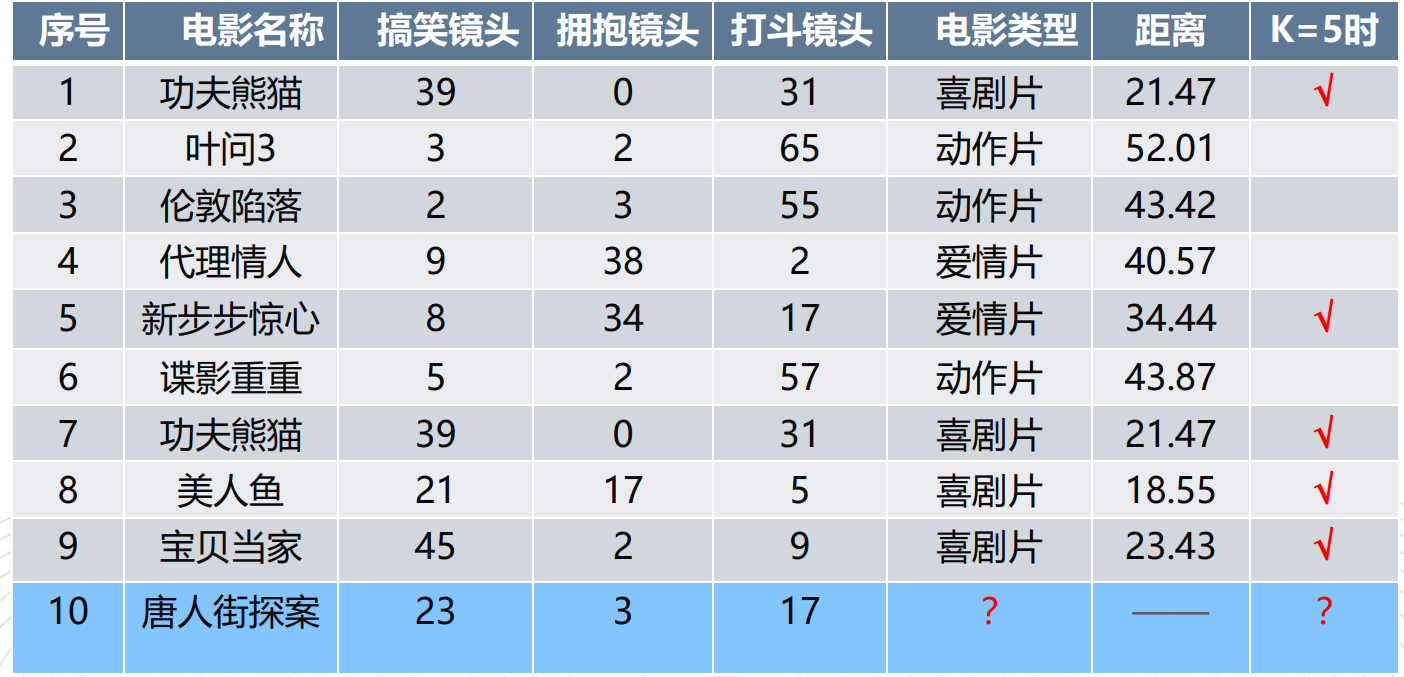import math

movie_data = {"宝贝当家": [45, 2, 9, "喜剧片"],
"美人鱼": [21, 17, 5, "喜剧片"],
"澳门风云3": [54, 9, 11, "喜剧片"],
"功夫熊猫3": [39, 0, 31, "喜剧片"],
"谍影重重": [5, 2, 57, "动作片"],
"叶问3": [3, 2, 65, "动作片"],
"伦敦陷落": [2, 3, 55, "动作片"],
"我的特工爷爷": [6, 4, 21, "动作片"],
"奔爱": [7, 46, 4, "爱情片"],
"夜孔雀": [9, 39, 8, "爱情片"],
"代理情人": [9, 38, 2, "爱情片"],
"新步步惊心": [8, 34, 17, "爱情片"]}

# 测试样本  唐人街探案": [23, 3, 17, "？片"]
#下面为求与数据集中所有数据的距离代码：
x = [23, 3, 17]
KNN = []
for key, v in movie_data.items():
d = math.sqrt((x - v) ** 2 + (x - v) ** 2 + (x - v) ** 2)
KNN.append([key, round(d, 2)])

# 输出所用电影到 唐人街探案的距离
print(KNN)

#按照距离大小进行递增排序
KNN.sort(key=lambda dis: dis)

#选取距离最小的k个样本，这里取k=5；
KNN=KNN[:5]
print(KNN)

#确定前k个样本所在类别出现的频率，并输出出现频率最高的类别
labels = {"喜剧片":0,"动作片":0,"爱情片":0}
for s in KNN:
label = movie_data[s]
labels[label] += 1
labels =sorted(labels.items(),key=lambda l: l,reverse=True)
print(labels,labels,sep='\n')

代码和例子来自：https://blog.csdn.net/saltriver/article/details/52502253
2-2 K值的选择
一、近似误差与估计误差：
近似误差：对现有训练集的训练误差，关注训练集，如果近似误差过小可能会出现过拟合的现象，对现有的训练集能有很好的预测，但是对未知的测试样本将会出现较大偏差的预测。模型本身不是最接近最佳模型。
估计误差：可以理解为对测试集的测试误差，关注测试集，估计误差小说明对未知数据的预测能力好，模型本身最接近最佳模型。
二、K值确定标准：
K值过小：k值小，特征空间被划分为更多子空间（模型的项越多），整体模型变复杂，容易发生过拟合，k值越小，选择的范围就比较小，训练的时候命中率较高，近似误差小，而用test的时候就容易出错，估计误差大，容易过拟合。
K值=N：无论输入实例是什么，都将简单的预测他属于训练实例中最多的类。
2-3 kd树
一、原理：
kd树(K-dimension tree)是一种对k维空间中的实例点进行存储以便对其进行快速检索的树形数据结构。 kd树是是一种二叉树，表示对k维空间的一个划分，构造kd树相当于不断地用垂直于坐标轴的超平面将K维空间切分，构成一系列的K维超矩形区域。kd树的每个结点对应于一个k维超矩形区域。 利用kd树可以省去对大部分数据点的搜索， 从而减少搜索的计算量。
一个三维k-d树。 第一次划分（红色）把根节点（白色）划分成两个节点，然后它们分别再次被划分（绿色） 为两个子节点。最后这四个子节点的每一个都被划分（蓝色） 为两个子节点。 因为没有更进一步的划分， 最后得到的八个节点称为叶子节点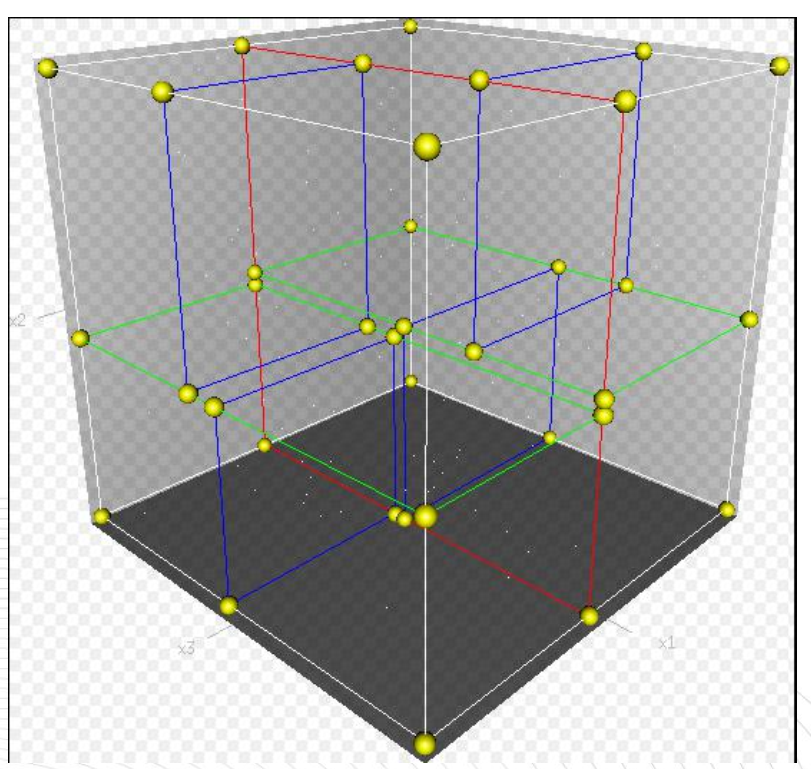类比“二分查找”： 给出一组数据： [9 1 4 7 2 5 0 3 8]， 要查找8。如果挨个查找（线性扫描），那么将会把数据集都遍历一遍。而如果排一下序那数据集就变成了： [0 1 2 3 4 5 6 7 8 9]， 按前一种方式我们进行了很多没有必要的查找， 现在如果我们以5为分界点， 那么数据集就被划分为了左右两个“簇” [0 1 2 3 4]和[6 7 8 9]。
因此， 根本久没有必要进入第一个簇，可以直接进入第二个簇进行查找。把二分查找中的数据点换成k维数据点， 这样的划分就变成了用超平面对k维空间的划分。空间划分就是对数据点进行分类， “挨得近”的数据点就在一个空间里面。
二、构造方法：
第一个问题简单的解决方法可以是选择随机选择某一维或按顺序选择，但是更好的方法应该是在数据比较分散的那一维进行划分（分散的程度可以根据方差来衡量）。好的划分方法可以使构建的树比较平衡， 可以每次选择中位数来进行划分， 这样问题2也得到了解决。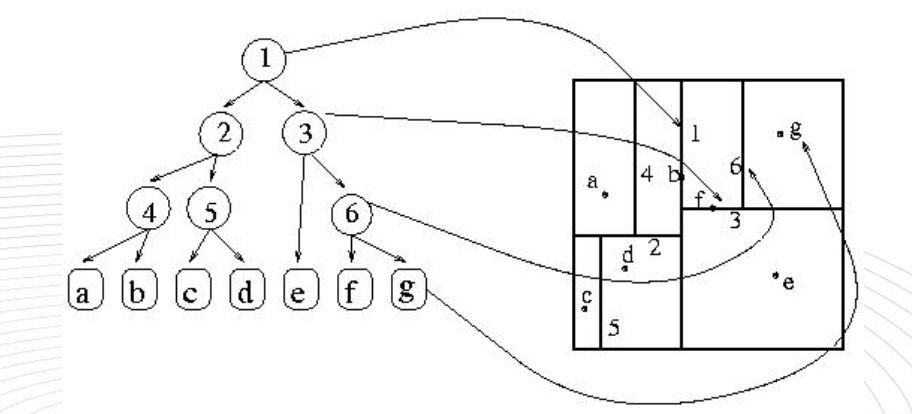代码实现：
# -*- coding: utf-8 -*-

#from operator import itemgetter
import sys
sys.setdefaultencoding('utf8')

# kd-tree每个结点中主要包含的数据结构如下
class KdNode(object):
def __init__(self, dom_elt, split, left, right):
self.dom_elt = dom_elt  # k维向量节点(k维空间中的一个样本点)
self.split = split      # 整数（进行分割维度的序号）
self.left = left        # 该结点分割超平面左子空间构成的kd-tree
self.right = right      # 该结点分割超平面右子空间构成的kd-tree

class KdTree(object):
def __init__(self, data):
k = len(data)  # 数据维度

def CreateNode(split, data_set): # 按第split维划分数据集exset创建KdNode
if not data_set:    # 数据集为空
return None
# key参数的值为一个函数，此函数只有一个参数且返回一个值用来进行比较
# operator模块提供的itemgetter函数用于获取对象的哪些维的数据，参数为需要获取的数据在对象中的序号
#data_set.sort(key=itemgetter(split)) # 按要进行分割的那一维数据排序
data_set.sort(key=lambda x: x[split])
split_pos = len(data_set) // 2      # //为Python中的整数除法
median = data_set[split_pos]        # 中位数分割点
split_next = (split + 1) % k        # cycle coordinates

# 递归的创建kd树
return KdNode(median, split,
CreateNode(split_next, data_set[:split_pos]),     # 创建左子树
CreateNode(split_next, data_set[split_pos + 1:])) # 创建右子树

self.root = CreateNode(0, data)         # 从第0维分量开始构建kd树,返回根节点

# KDTree的前序遍历
def preorder(root):
print root.dom_elt
if root.left:      # 节点不为空
preorder(root.left)
if root.right:
preorder(root.right)

if __name__ == "__main__":
data = [[2,3],[5,4],[9,6],[4,7],[8,1],[7,2]]
kd = KdTree(data)
preorder(kd.root)



展开全文• @[TOC]《机器学习实战》-KNN算法心得) 准备开始在CSDN上用博客记录学习心得，同时也分享一些其中自己产生的脑洞（想法） PS：第一次在CSDN上写，还不习惯这个编写方式 在写之前说一下，因为书里很多代码在python3...
@[TOC]《机器学习实战》-KNN算法心得)
准备开始在CSDN上用博客记录学习心得，同时也分享一些其中自己产生的脑洞（想法）
PS：第一次在CSDN上写，还不习惯这个编写方式
在写之前说一下，因为书里很多代码在python3以后都会编译错误，所以关于《机器学习实战》的总结，不会具体分析代码，代码问题在overstackflow或者matplotlib官网都能搜索到。
KNN是处理分类问题的，而且是监督的，即需要预先认为的给出一些已知类别的数据供KNN计算。其中对一个物体，需要人为的分成多个属性，并求出每个属性的值。它对属性的值要求可以是连续也可以是离散的。
书里介绍的KNN算法算是全书中最好理解的一部分了。其核心理论是将类别的多个属性看做是多维空间的参数，将已经识别的类数据库根据属性的值放置在这个多维空间中，然后用未知类别物体的属性值与已知的各个点进行距离计算（sqrt（x12+x22+x3^2……）），离得最近的点的类别就是这个未知类别物体的类别，当然，考虑到可能存在的误差，它取了前K个最近距离点，统计其中出现的类别次数，并返回出现次数最多的类别。
关于空间距离的说法，在很多机器学习的论文里，都会提到多维或者超维或者降维的问题，因为当初学线性代数的时候一上来就是行列式，所以以前没理解，后来看了一本《introduce to Linear algebra》（强烈推荐），上面一开始介绍时将[1,2,3]T介绍为三维空间中的指向从（0,0,0）点指向(1,2,3)的一个向量，因此上文所说的多维空间距离就是因为KNN把每个属性看做一个坐标系，值就是这个类别点在这个坐标系上的坐标，才有了距离一说。
另外，因为每个属性的值有大有小，所以在数据上预先将所以数据按照：（对应属性的值-此属性的最小值）/（此属性的最大值-最小值）的方式都归一化到[0,1]的范围内。
流程如下图所示：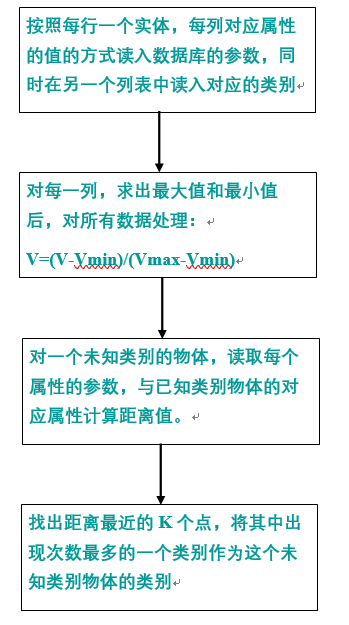按照KNN的算法，可以进行书中提供数据的分类，有意思的是，改变K值会发现，K值的提高并不会降低分类的错误率（错误率=错误的分类/总的数据，这是用一些检测数据来计算的），反而可能增加错误率，这是因为KNN不是一种特征提取的算法，它并不会从数据中识别出那些核心分类的特征，而只是单纯的计算用人为给出的属性值组成的参数距离。
同时减少K值对KNN的算法时间并没有明显的减少，从上面的算法就可以看出，找出前K个数，在K较小时并不耗时（如果不按书里的算法（先全排序再取前K个数）而是堆排后弹出前K个值还会更省时，而且因为其实前K个数不需要排序，所以用快排里的取前K的算法甚至在O（N）时间里就得到），反而是需要对已知分类的N个数，都要计算空间距离，相当于对矩阵进行了做差后平方（一次全遍历矩阵，O（NN）），求和（一次全遍历矩阵，O（NN）），开根号（因为是列表，所以是O（N）），当数据较大后非常耗时（都是浮点计算），数据小的时候又不准确，所以KNN是个非常耗时的算法。
另外，个人分析，KNN将属性值视作坐标系的做法，相当于默认属性间线性无关，如果人为选择的属性间线性相关（即相互影响），真实的距离和计算的距离就有偏差了，可能计算出来是最近的点，实际一旦正交化距离就远了。
KNN算法部分就到这里，接下来是决策树。


展开全文机器学习实战
• KNN算法简介 KNN（K-Nearest Neighbor）最邻近分类算法是数据挖掘分类（classification）技术中最简单的算法之一，其指导思想是”近朱者赤，近墨者黑“，即由你的邻居来推断出你的类别。 KNN最邻近分类算法的...
KNN算法简介

KNN（K-Nearest Neighbor）最邻近分类算法是数据挖掘分类（classification）技术中最简单的算法之一，其指导思想是”近朱者赤，近墨者黑“，即由你的邻居来推断出你的类别。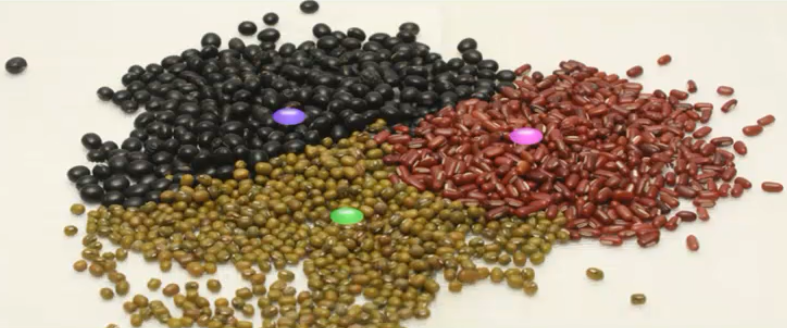KNN最邻近分类算法的实现原理：为了判断未知样本的类别，以所有已知类别的样本作为参照，计算未知样本与所有已知样本的距离，从中选取与未知样本距离最近的K个已知样本，根据少数服从多数的投票法则（majority-voting），将未知样本与K个最邻近样本中所属类别占比较多的归为一类。

以上就是KNN算法在分类任务中的基本原理，实际上K这个字母的含义就是要选取的最邻近样本实例的个数，在 scikit-learn 中 KNN算法的 K 值是通过 n_neighbors 参数来调节的，默认值是 5。

如下图所示，如何判断绿色圆应该属于哪一类，是属于红色三角形还是属于蓝色四方形？如果K=3，由于红色三角形所占比例为2/3，绿色圆将被判定为属于红色三角形那个类，如果K=5，由于蓝色四方形比例为3/5，因此绿色圆将被判定为属于蓝色四方形类。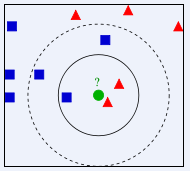由于KNN最邻近分类算法在分类决策时只依据最邻近的一个或者几个样本的类别来决定待分类样本所属的类别，而不是靠判别类域的方法来确定所属类别的，因此对于类域的交叉或重叠较多的待分样本集来说，KNN方法较其他方法更为适合。

KNN算法的关键：

(1) 样本的所有特征都要做可比较的量化

若是样本特征中存在非数值的类型，必须采取手段将其量化为数值。例如样本特征中包含颜色，可通过将颜色转换为灰度值来实现距离计算。

(2) 样本特征要做归一化处理

样本有多个参数，每一个参数都有自己的定义域和取值范围，他们对距离计算的影响不一样，如取值较大的影响力会盖过取值较小的参数。所以样本参数必须做一些 scale 处理，最简单的方式就是所有特征的数值都采取归一化处置。

(3) 需要一个距离函数以计算两个样本之间的距离

通常使用的距离函数有：欧氏距离、余弦距离、汉明距离、曼哈顿距离等，一般选欧氏距离作为距离度量，但是这是只适用于连续变量。在文本分类这种非连续变量情况下，汉明距离可以用来作为度量。通常情况下，如果运用一些特殊的算法来计算度量的话，K近邻分类精度可显著提高，如运用大边缘最近邻法或者近邻成分分析法。

以计算二维空间中的A(x1,y1)、B(x2,y2)两点之间的距离为例，欧氏距离和曼哈顿距离的计算方法如下图所示：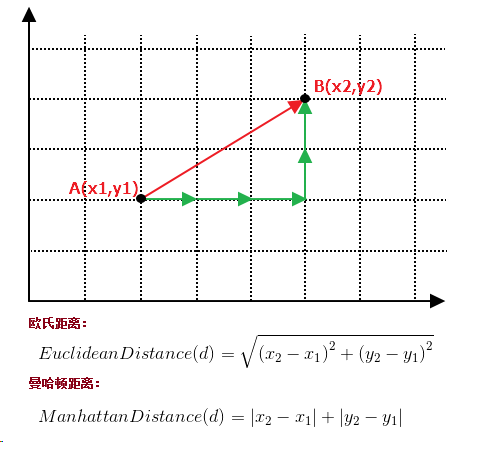(4) 确定K的值

K值选的太大易引起欠拟合，太小容易过拟合，需交叉验证确定K值。

KNN算法的优点：

1.简单，易于理解，易于实现，无需估计参数，无需训练；

2. 适合对稀有事件进行分类；

3.特别适合于多分类问题(multi-modal,对象具有多个类别标签)， kNN比SVM的表现要好。

KNN算法的缺点：

KNN算法在分类时有个主要的不足是，当样本不平衡时，如一个类的样本容量很大，而其他类样本容量很小时，有可能导致当输入一个新样本时，该样本的K个邻居中大容量类的样本占多数，如下图所示。该算法只计算最近的邻居样本，某一类的样本数量很大，那么或者这类样本并不接近目标样本，或者这类样本很靠近目标样本。无论怎样，数量并不能影响运行结果。可以采用权值的方法(和该样本距离小的邻居权值大)来改进。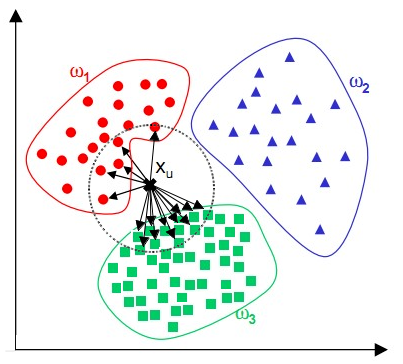该方法的另一个不足之处是计算量较大，因为对每一个待分类的文本都要计算它到全体已知样本的距离，才能求得它的K个最近邻点。

可理解性差，无法给出像决策树那样的规则。

KNN算法实现

要自己动手实现KNN算法其实不难，主要有以下三个步骤：

算距离：给定待分类样本，计算它与已分类样本中的每个样本的距离；

找邻居：圈定与待分类样本距离最近的K个已分类样本，作为待分类样本的近邻；

做分类：根据这K个近邻中的大部分样本所属的类别来决定待分类样本该属于哪个分类；

以下是使用Python实现KNN算法的简单示例：

import math
import csv
import operator
import random
import numpy as np
from sklearn.datasets import make_blobs

#Python version 3.6.5

# 生成样本数据集 samples(样本数量) features(特征向量的维度) centers(类别个数)
def createDataSet(samples=100, features=2, centers=2):
return make_blobs(n_samples=samples, n_features=features, centers=centers, cluster_std=1.0, random_state=8)

# 加载鸢尾花卉数据集 filename(数据集文件存放路径)
with open(filename, 'rt') as csvfile:
dataset = list(lines)
for x in range(len(dataset)):
for y in range(4):
dataset[x][y] = float(dataset[x][y])
return dataset

# 拆分数据集 dataset(要拆分的数据集) split(训练集所占比例) trainingSet(训练集) testSet(测试集)
def splitDataSet(dataSet, split, trainingSet=[], testSet=[]):
for x in range(len(dataSet)):
if random.random() <= split:
trainingSet.append(dataSet[x])
else:
testSet.append(dataSet[x])
# 计算欧氏距离
def euclideanDistance(instance1, instance2, length):
distance = 0
for x in range(length):
distance += pow((instance1[x] - instance2[x]), 2)
return math.sqrt(distance)

# 选取距离最近的K个实例
def getNeighbors(trainingSet, testInstance, k):
distances = []
length = len(testInstance) - 1
for x in range(len(trainingSet)):
dist = euclideanDistance(testInstance, trainingSet[x], length)
distances.append((trainingSet[x], dist))
distances.sort(key=operator.itemgetter(1))

neighbors = []
for x in range(k):
neighbors.append(distances[x])
return neighbors

#  获取距离最近的K个实例中占比例较大的分类
def getResponse(neighbors):
for x in range(len(neighbors)):
response = neighbors[x][-1]
else:

# 计算准确率
def getAccuracy(testSet, predictions):
correct = 0
for x in range(len(testSet)):
if testSet[x][-1] == predictions[x]:
correct += 1
return (correct / float(len(testSet))) * 100.0

def main():
# 使用自定义创建的数据集进行分类
# x,y = createDataSet(features=2)
# dataSet= np.c_[x,y]

# 使用鸢尾花卉数据集进行分类

print(dataSet)
trainingSet = []
testSet = []
splitDataSet(dataSet, 0.75, trainingSet, testSet)
print('Train set:' + repr(len(trainingSet)))
print('Test set:' + repr(len(testSet)))
predictions = []
k = 7
for x in range(len(testSet)):
neighbors = getNeighbors(trainingSet, testSet[x], k)
result = getResponse(neighbors)
predictions.append(result)
print('>predicted=' + repr(result) + ',actual=' + repr(testSet[x][-1]))
accuracy = getAccuracy(testSet, predictions)
print('Accuracy: ' + repr(accuracy) + '%')
main()



尾花卉数据文件百度网盘下载链接：https://pan.baidu.com/s/10vI5p_QuM7esc-jkar2zdQ 密码：4und

KNN算法应用

使用KNN算法处理简单分类任务

在scikit-learn中，内置了若干个玩具数据集（Toy Datasets），还有一些API让我们可以自己动手生成一些数据集。接下来我们将使用scikit-learn的make_blobs函数来生成一个样本数量为200，分类数量为2的数据集，并使用KNN算法来对其进行分类。

# 导入画图工具
import matplotlib.pyplot as plt
# 导入数组工具
import numpy as np
# 导入数据集生成器
from sklearn.datasets import make_blobs
# 导入KNN 分类器
from sklearn.neighbors import KNeighborsClassifier
# 导入数据集拆分工具
from sklearn.model_selection import train_test_split

# 生成样本数为200，分类数为2的数据集
data=make_blobs(n_samples=200, n_features=2,centers=2, cluster_std=1.0, random_state=8)
X,Y=data

# 将生成的数据集进行可视化
# plt.scatter(X[:,0], X[:,1],s=80, c=Y,  cmap=plt.cm.spring, edgecolors='k')
# plt.show()

clf = KNeighborsClassifier()
clf.fit(X,Y)

# 绘制图形
x_min,x_max=X[:,0].min()-1,X[:,0].max()+1
y_min,y_max=X[:,1].min()-1,X[:,1].max()+1
xx,yy=np.meshgrid(np.arange(x_min,x_max,.02),np.arange(y_min,y_max,.02))
z=clf.predict(np.c_[xx.ravel(),yy.ravel()])

z=z.reshape(xx.shape)
plt.pcolormesh(xx,yy,z,cmap=plt.cm.Pastel1)
plt.scatter(X[:,0], X[:,1],s=80, c=Y,  cmap=plt.cm.spring, edgecolors='k')
plt.xlim(xx.min(),xx.max())
plt.ylim(yy.min(),yy.max())
plt.title("Classifier:KNN")

# 把待分类的数据点用五星表示出来
plt.scatter(6.75,4.82,marker='*',c='red',s=200)

# 对待分类的数据点的分类进行判断
res = clf.predict([[6.75,4.82]])
plt.text(6.9,4.5,'Classification flag: '+str(res))

plt.show()

程序执行后得到结果如下图所示：使用KNN算法处理多元分类任务

接下来，我们再使用scikit-learn的make_blobs函数来生成一个样本数量为500，分类数量为5的数据集，并使用KNN算法来对其进行分类。

# 导入画图工具
import matplotlib.pyplot as plt
# 导入数组工具
import numpy as np
# 导入数据集生成器
from sklearn.datasets import make_blobs
# 导入KNN 分类器
from sklearn.neighbors import KNeighborsClassifier
# 导入数据集拆分工具
from sklearn.model_selection import train_test_split

# 生成样本数为500，分类数为5的数据集
data=make_blobs(n_samples=500, n_features=2,centers=5, cluster_std=1.0, random_state=8)
X,Y=data

# 将生成的数据集进行可视化
# plt.scatter(X[:,0], X[:,1],s=80, c=Y,  cmap=plt.cm.spring, edgecolors='k')
# plt.show()

clf = KNeighborsClassifier()
clf.fit(X,Y)

# 绘制图形
x_min,x_max=X[:,0].min()-1,X[:,0].max()+1
y_min,y_max=X[:,1].min()-1,X[:,1].max()+1
xx,yy=np.meshgrid(np.arange(x_min,x_max,.02),np.arange(y_min,y_max,.02))
z=clf.predict(np.c_[xx.ravel(),yy.ravel()])

z=z.reshape(xx.shape)
plt.pcolormesh(xx,yy,z,cmap=plt.cm.Pastel1)
plt.scatter(X[:,0], X[:,1],s=80, c=Y,  cmap=plt.cm.spring, edgecolors='k')
plt.xlim(xx.min(),xx.max())
plt.ylim(yy.min(),yy.max())
plt.title("Classifier:KNN")

# 把待分类的数据点用五星表示出来
plt.scatter(0,5,marker='*',c='red',s=200)

# 对待分类的数据点的分类进行判断
res = clf.predict([[0,5]])
plt.text(0.2,4.6,'Classification flag: '+str(res))
plt.text(3.75,-13,'Model accuracy: {:.2f}'.format(clf.score(X, Y)))

plt.show()

程序执行后得到结果如下图所示：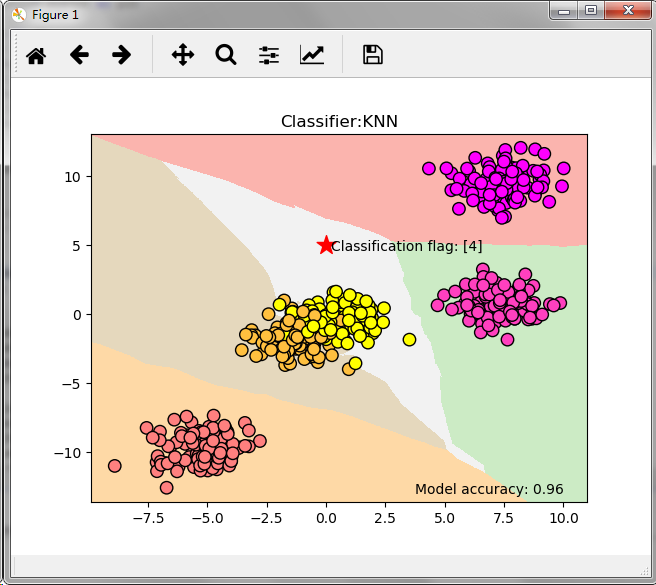使用KNN算法进行回归分析

这里我们使用scikit-learn的make_regression生成数据集来进行实验，演示KNN算法在回归分析中的表现。

# 导入画图工具
import matplotlib.pyplot as plt
# 导入数组工具
import numpy as np

# 导入用于回归分析的KNN模型
from sklearn.neighbors import KNeighborsRegressor
# 导入数据集拆分工具
from sklearn.model_selection import train_test_split
# 导入数据集生成器
from sklearn.datasets.samples_generator import make_regression
from docutils.utils.math.math2html import LineWriter

# 生成样本数为200，分类数为2的数据集
X,Y=make_regression(n_samples=100,n_features=1,n_informative=1,noise=50,random_state=8)

# 将生成的数据集进行可视化
# plt.scatter(X,Y,s=80, c='orange',  cmap=plt.cm.spring, edgecolors='k')
# plt.show()
reg = KNeighborsRegressor(n_neighbors=5)

reg.fit(X,Y)

# 将预测结果用图像进行可视化
z = np.linspace(-3,3,200).reshape(-1,1)
plt.scatter(X,Y,c='orange',edgecolor='k')
plt.plot(z,reg.predict(z),c='k',Linewidth=3)
#
plt.title("KNN Regressor")

plt.show()

程序执行后得到结果如下图所示：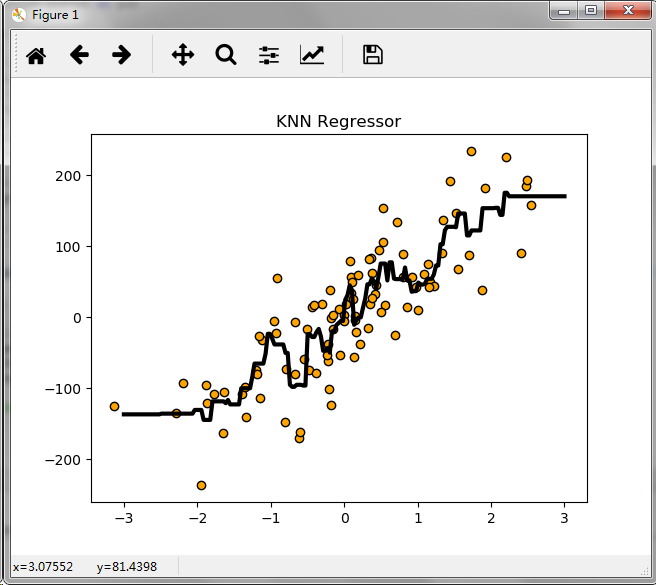KNN算法项目实战----酒的分类

from sklearn.datasets.base import load_wine
from sklearn.model_selection import train_test_split
from sklearn.neighbors import KNeighborsClassifier
import numpy as np

# 从 sklearn的datasets模块载入数据集加载酒的数据集
print(wineDataSet)
print("红酒数据集中的键：\n{}".format(wineDataSet.keys()))
print("数据概况：\n{}".format(wineDataSet['data'].shape))
print(wineDataSet['DESCR'])

# 将数据集拆分为训练数据集和测试数据集
X_train,X_test,y_train,y_test=train_test_split(wineDataSet['data'],wineDataSet['target'],random_state=0)
print("X_train shape:{}".format(X_train.shape))
print("X_test shape:{}".format(X_test.shape))
print("y_train shape:{}".format(y_train.shape))
print("y_test shape:{}".format(y_test.shape))

knn = KNeighborsClassifier(n_neighbors=1)
knn.fit(X_train,y_train)
print(knn)

# 评估模型的准确率
print('测试数据集得分：{:.2f}'.format(knn.score(X_test,y_test)))

# 使用建好的模型对新酒进行分类预测
X_new = np.array([[13.2,2.77,2.51,18.5,96.6,1.04,2.55,0.57,1.47,6.2,1.05,3.33,820]])
prediction = knn.predict(X_new)
print("预测新酒的分类为：{}".format(wineDataSet['target_names'][prediction]))

执行程序后打印如下结果：

X_train shape:(133, 13)
X_test shape:(45, 13)
y_train shape:(133,)
y_test shape:(45,)
KNeighborsClassifier(algorithm='auto', leaf_size=30, metric='minkowski',
metric_params=None, n_jobs=1, n_neighbors=1, p=2,
weights='uniform')
测试数据集得分：0.76
预测新酒的分类为：['class_2']

参考书籍：《深入浅出 Python 机器学习》 作者：段小手

展开全文最邻近算法 python
• 机器学习之KNN算法机器学习之KNN算法 原理 伪代码 Python 代码实现原理KNN的原理非常简单，在训练样本集中，知道每个数据的标签，那么输入未标注的新数据时，将新数据的每个特征与训练样本数据对应的特征进行比较，...
机器学习之KNN算法

机器学习之KNN算法原理
伪代码
Python 代码实现

原理

KNN的原理非常简单，在训练样本集中，知道每个数据的标签，那么输入未标注的新数据时，将新数据的每个特征与训练样本数据对应的特征进行比较，然后算法提取样本集中特征最相似的数据（最近邻）的分类标签，作为新数据的标签。
一般来说，我们选择样本数据集中前K个（一般不大于20）最相似的数据（这就是K-近邻的出处），选择K个最相似数据中出现次数最多的分类标签，作为新数据的分类标签。


伪代码

1、计算已知类别属性的数据中的点与当前需要预测点之恋的距离；
2、按照距离递增次序排列（距离越小，越相似）；
3、选择与当前点距离最小的K个点；
4、确定前K个点所在类别出现的频率；
5、返回前K个点出现频率最高的类别作为当前点的预测分类；

Python 代码实现

K-近邻算法  python3.6
def classify0(inX,dataSet, labels ,k):
dataSetSize = dataSet.shape   #shape 是numpy数据库中的函数，用于计算矩阵的行和列，代替行数，代表列数
diffMat = tile(inX, (dataSetSize,1)) - dataSet  #tile(A,rep)函数为重复A的各个维度,rep重复的次数
sqDiffMat = diffMat ** 2
sqDistances = sqDiffMat.sum(axis = 1)
distances = sqDistances **0.5
sortedDistIndicies = distances.argsort()  #  计算两个数据间的欧式距离
classCount = {}
for i in range(k):
voteIlabel = labels[sortedDistIndicies[i]]
classCount[voteIlabel] = classCount.get(voteIlabel, 0 ) +1
sortedClassCount = sorted( classCount.items(), key = operator.itemgetter(1) , reverse =True)
# classCount.items()将字典分解为元祖列表，
# operator.itemgetter(1) 代表获取对象的第一个域的值
return sortedClassCount 

处理不同取值范围的特征值时，需要进行归一化处理  def autoNorm(dataSet):
minVals = dataSet.min(0)                    #最小特征值
maxVals = dataSet.max(0)                    #最大特征值
ranges = maxVals - minVals                  #特征值变化范围
normDataSet = zeros(shape(dataSet))
m = dataSet.shape
normDataSet = dataSet - tile(minVals, (m,1))
normDataSet = normDataSet/tile(ranges, (m,1))   #element wise divide，归一化特征值矩阵
return normDataSet, ranges, minVals 
展开全文机器学习
• ok,今天花了一天时间看了小人书(机器学习实战),并且撸到了KNN算法,并完成了一个KNN算法的应用 真的!!!小人书是本特别不错的适合入门ML的书!!!!! 没有繁杂的数学推导过程,先给出概念和实战应用,等对ML有个大致了解...
• KNN（最邻近规则分类K-Nearest-Neighibor)KNN算法 1. 综述 1.1 Cover和Hart在1968年提出了最初的邻近算法 1.2 分类(classification)算法 1.3 输入基于实例的学习(instance-based learning), 懒惰学习(lazy ...
• 目录机器学习实战之KNN算法（代码）详解KNN算法一般流程算法的一般操作创建数据集KNN算法代码详解代码测试运行实现： 机器学习实战之KNN算法（代码）详解 KNN算法一般流程       &...机器学习
• 机器学习—无监督学习-KNN算法 1.KNN算法是什么？ KNN算法是一种监督式学习算法，核心思想是用距离最近的k个样本数据的分类来代表目标数据的分类。从原理上来说， 存在一个训练样本集，这个数据训练样本的数据集合中...
• 介绍机器学习算法KNN，并提供matlab，python实例，提供手写识别系统matlab完整代码机器学习 matlab python
• 机器学习实战：KNN算法讲解  KNN算法本章内容来至于《统计学习与方法》李航,《机器学习》周志华,以及《机器学习实战》Peter HarringTon,相互学习,不足之处请大家多多指教  1.1 KNN算法的优缺点  1.2 KNN...机器学习 统计学
• KNN算法学习总结 一、实验准备 1、实验内容和目的 使用KNN算法预测鸢尾花的种类，其中训练数据集为train.txt，测试集为test.txt。训练样本为120个，测试样本为30个。 2、实验原理 K最近邻(k-NearestNeighbor, ...
• 看到这个名字，是不是觉得很高大上？ 看到网上的那些大佬写了一堆的代码，一堆的分析，是不是有点头昏脑胀？ 这还是机器学习中最简单的算法么？...相信短短的20行代码就会让你快速掌握Knn算法(机器学习中最python 机器学习 算法
• 所有代码请移步GitHub——kNNbyPython 很多人在第一次听到机器学习的时候都不知所措，无从下手。起初我也是这样的，各种看别人的博客，吴恩达的课程也...KNN算法应该是机器学习中最简单的算法之一，作为机器学习...算法 机器学习
• 1、KNN算法概论　kNN算法的核心思想是：如果一个样本在特征空间中的k个最相邻的样本中的大多数属于某一个类别，则该样本也属于这个类别，并具有这个类别上样本的特性。该方法在确定分类决策上只依据最邻近的一个或者...机器学习 十大经典机器学习算法 近邻算法
• 一、KNN算法概述： 1.KNN算法的工作原理是： （1）存在一个训练样本集，并且知道样本集中每一数据与所属分类的对应关系，即每个数据都存在分类标签。 （2）若此时输入不带标签的新数据之后，将新数据的每个特征与...机器学习 学习笔记
• 用python学习机器学习的笔记，所有的代码和实例来源于《机器学习实战》一书。所有源代码和数据都可以在我的github上下载。 1.机器学习基础 机器学习可以分为监督学习和无监督学习，监督学习又可以分为分类...knn算法 机器学习 人工智能 算法
• KNN（K-Nearest Neighbor）算法是机器学习算法中最基础、最简单的算法之一。它既能用于分类，也能用于回归...KNN算法是一种非常特别的机器学习算法，因为它没有一般意义上的学习过程。它的工作原理是利用训练数据...人工智能 机器学习 KNN算法 回归预测
• KNN可以说是最简单的分类算法之一，同时，它也是最常用的分类算法之一，注意KNN算法是有监督学习中的分类算法，它看起来和另一个机器学习算法Kmeans有点像（Kmeans是无监督学习算法），但却是有本质区别的。...
• 机器学习KNN算法基本原理与python实现 k-近邻算法(k-Nearest Neighbour algorithm),又称为KNN算法,是数据挖掘技术中原理最简单的算法。KNN 的工作原理:给定一个已知标签类别的训练数据集,输入没有标签的新数据后,在...
• 机器学习算法knn课件，机器学习算法knn课件，机器学习算法knn课件。
...